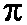# Pi Approximation DayDid you miss Pi Day on March 14? Well, math lovers have a second chance to celebrate today!

Pi Day is held on March 14 (which is 3/14 in the month/day format commonly used in the US) . The numbers 3, 1, and 4 are the three most significant digits of π in the decimal form 3.14.

Pi Approximation Day is held on July 22 (which is 22/7 in the day/month format common in other countries). The fraction 22/7 is a common approximation of π, since the number 22 divided by 7 equals 3.14.

Pi oris a number, approximately equal to 3.14, that represents the ratio of a circle’s circumference to its diameter. Pi appears as an important constant in many mathematical equations, and it’s the most recognized mathematical constant in the world.

Check out these links: Pi Facts | Pi Fun | Pi Day Activities

This entry was posted in 500 Pure Science and tagged , , , . Bookmark the permalink.